# Some terms & definitions. Angles, angle pairs, angle measure

Topic:
Angles, Terms
A series of applets about some terms/definitions. Angle classification, angle measurement with a protractor, angle pair relationships, sum of interior angles. Interior and exterior angles of a triangle
•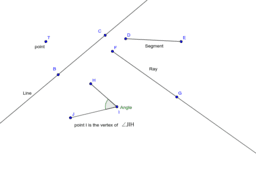### Basic Geometric figures - illustration & 4 questions

•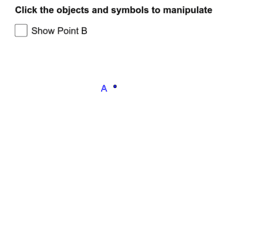### Point(s), a Line, Ray(s), Segment(s), & an angle - interactive & 3 questions

•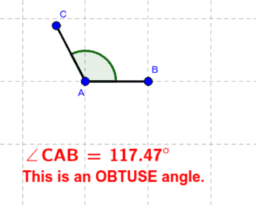### Types of angles - the 5 classifications

•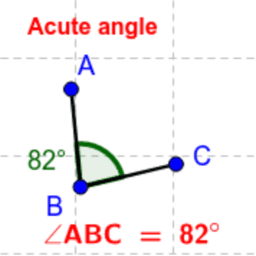### Animated acute angles

•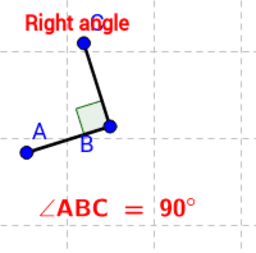### Animated view of right angles.

•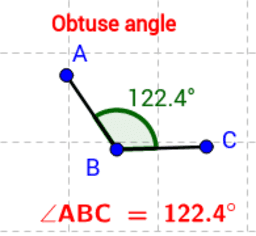### Animated view of obtuse angles.

•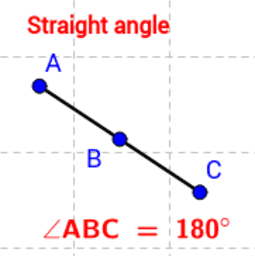### Animated view of straight angles.

•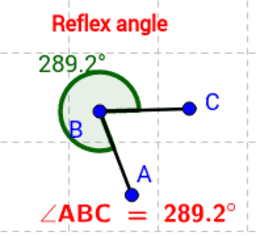### Animated view of reflex angles.

•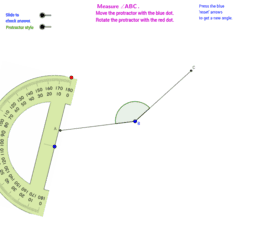### Protractor Practice (Random)

•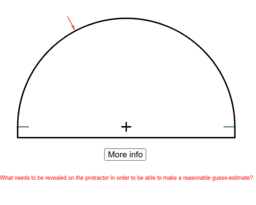### "Broken" Protractor

•### Protractor Practice (Not Random)

•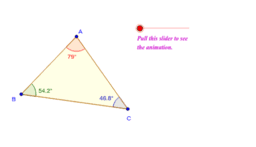### "proof" of the triangle sum theorem

•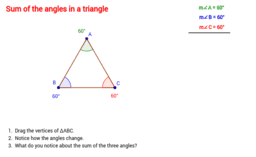### Sum of the interior angles in a triangle is always _

•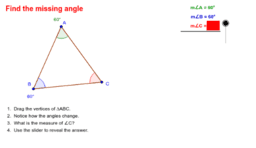### Missing angle measure in a triangle

•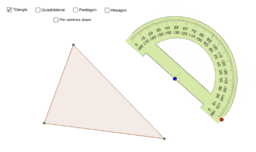### Sum of the interior angles of a polygon

•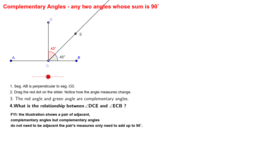### Definition of complementary angles

•### Missing complementary angle measure

•### Supplementary angles - a pair of angles whose sum is always _

•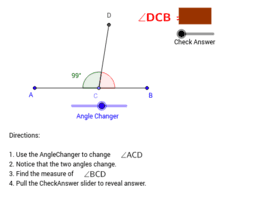### What is the measure of an angle's supplement?

•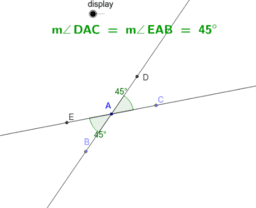### Vertical angles

•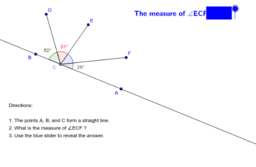### Angles on one side of a line - parts of a straight angle

•